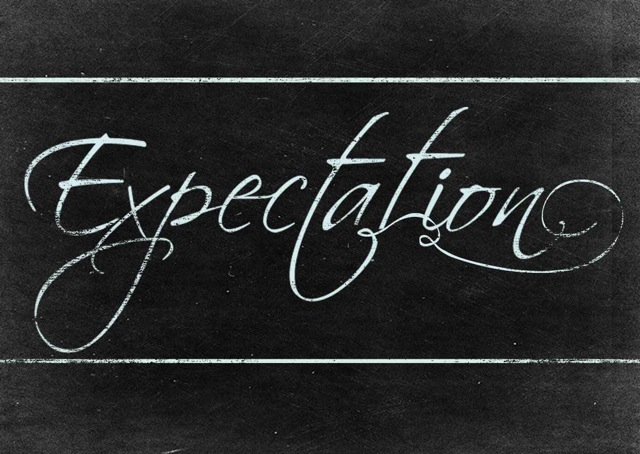## Intro

In here I will set a notation of the mathematical expectation of discrete and continuous Random Variable (RV).

The idea is to outline some of the most important notations, properties and rules.

## Random experiment

Random experiment is modeled by a probability space $(\Omega, \mathscr F, P)$.

$\Omega$ is the set of outcomes, $\mathscr F$ the collection of events, and $P$ the probability measure on the sample space $(\Omega, \mathscr F)$.

## Random Variable

$X$ is a random variable for the experiment, taking values in $S \subseteq \mathbb R$. We say a random variable is a product of the random experiment that has associated probability distribution.

## Expected value

In probability theory, the expected value of a random variable $X$ denoted as $\mathbb{E}(X)$ is generalization of the weighted average, and is intuitively the arithmetic mean of a large number of independent realizations of $X$.

Synonym names:

• mean
• average
• first moment
• center of distribution
• expected value of random variable

By taking the expected value of various functions of a general random variable, we can measure many interesting features of its distribution, including spread, skewness, kurtosis, and correlation.

### Discrete Random Variable Expectation

In case of the discrete random variable $X$ the expectation, or expected is:

\begin{aligned} \mathbb{E}(X)=\sum_{x \in S} x P(x)\end{aligned}

where $P$ is the probability density function PDF.

### Continuous Random Variable Expectation

In case $X$ has continuous distribution:

\begin{aligned}\mathbb{E}(X)=\int_{S} x \ P(x) d x\end{aligned}

$X$ may have mixed discrete and continuous distribution

### General discrete RV rewrite

In general case we may write:

\begin{aligned} \mathbb{E}_{X \sim P} [ f(X) ]= \sum_{x} P(x) f(x) \end{aligned}

$X \sim P$ means $X$ is drawn from distribution $P(x)$ or just from $P$.

Where $Y = f(X)$ is also a random variable.

The last is known as change of variables theorem.

This enables us to compute $\mathbb{E}_{X \sim P} [ f(X) ]$ without having to first find the probability density function $f(X)$.

### General continuous RV rewrite

For continuous variables we compute the integral:

\begin{aligned} \mathbb{E}_{X \sim P}[f(X)]=\int_{S} P(x) f(x) d x\end{aligned}

## Properties

### Linearity

There are two simple rules

addition: $\mathbb E(X + Y) = \mathbb E(X) + \mathbb E(Y)$

scalding: $\mathbb{E}(c X)=c \ \mathbb{E}(X)$

That help us form:

$\mathbb E[\alpha X+\beta Y]=\alpha \ \mathbb E[X]+\beta \ \mathbb E[Y]$

Linearity of expectation is the property that the expected value of the sum of random variables is equal to the sum of their individual expected values.

Note that if we scale the random variable $X$ the mean will also be scaled. If in the previous case the mean of $X$ was 1, $\mathbb E[X]=1$, then the mean of $\alpha X$ will be $\alpha$, $\mathbb E[\alpha X]=\alpha$.

### Symmetry

If $X$ has a distribution that is symmetric about $a \in \mathbb{R}$, then $\mathbb{E}(X)=a$.

Proof:

$\mathbb{E}(a-X)=\mathbb{E}(X-a)$ so by linearity

$a-\mathbb{E}(X)=\mathbb{E}(X)-a .$

$\mathbb{E}(X)= a$

### Independence

If $X$ and $Y$ are independent real-valued random variables then $\mathbb{E}(X Y)=\mathbb{E}(X) \mathbb{E}(Y)$

## Variance

Variance gives us the measure how much values of a function of a random variable $X$ vary as we sample different values of $X$ from it’s probability distribution.

$\operatorname{var}(f(X))=\mathbb{E}\left[(f(X)-\mathbb{E}[f(X)])^{2}\right]$

Or simple:

$\operatorname{var}(X)=\mathbb{E}\left[(X-\mathbb{E}(X))^{2}\right]$

Or even simpler:

$\operatorname{var}(X)=\mathbb{E}\left(X^{2}\right)-[\mathbb{E}(X)]^{2}$

## Standard Deviation

We can simple define it via Variance:

$\sigma = \operatorname{sd}(X)=\sqrt{\operatorname{var}(X)}$

## Covariance

The covariance gives some sense of how much two values are linearly related to each other, as well as the scale of these variables:

$\operatorname{cov}(X, Y)=\mathbb{E}([X-\mathbb{E}(X)][Y-\mathbb{E}(Y)])$

or if we use change of variables theorem:

$\operatorname{cov}\left(f(X), g(Y)\right)=\mathbb{E}[(f(X)-\mathbb{E}[f(X)])(g(Y)-\mathbb{E}[g(Y)])]$

### Properties of covariance:

Joint rule

$\operatorname{cov}(X, Y)=\mathbb{E}(X Y)-\mathbb{E}(X) \mathbb{E}(Y)$

When $X$ and $Y$ are not correlated:

$\operatorname{cov}(X, Y)=0$

$\mathbb{E}(X Y) =\mathbb{E}(X) \mathbb{E}(Y)$

Symmetry

$\operatorname{cov}(X, Y)=\operatorname{cov}(Y, X)$

We can use it to define variance

$\operatorname{cov}(X, X)=\operatorname{var}(X)$

## Correlation

To define correlation we use the fact we need to normalize the covariance:

\begin{aligned} \operatorname{cor}(X, Y)= \frac{\operatorname{cov}(X, Y)}{\operatorname{sd}(X) \operatorname{sd}(Y)} \end{aligned}

## Moments greater than 2

Third moment also known as skewness:

$skw(X) = \mathbb E\left[\left(\frac{X - \mu}{\sigma}\right)^3\right]$

Fourth moment kurtosis:

$kur(X) = \mathbb E\left[\left(\frac{X - \mu}{\sigma}\right)^4\right]$

## Examples

If $a \in \mathbb{R}$, $X$ and $Y$ are continuous random variables and $p(x)$ is the probability density function.

Using the above definitions:

\begin{aligned} \mathbb{E}[X] &=\int_{-\infty}^{\infty} x p(x) d x = \mu_X \\ \operatorname{var}(X) &=\mathbb{E}\left[(X-\mathbb{E}[X])^{2}\right] = \sigma_X^2\end{aligned}

Derive the expectation and variance of the following random variables in terms of $\mathbb{E}[X], \mathbb{E}[Y], \operatorname{var}(X)$ and $\operatorname{var}(Y)$ :

a) $X+a$

b) $a X$

c) $X+Y$

Derivations:

a)

\begin{aligned} \mathbb{E}[X+a] &=\int_{-\infty}^{\infty}(x+a) p(x) d x \\ &=\int_{-\infty}^{\infty} x p(x) d x+a \int_{-\infty}^{\infty} p(x) d x \\ &=\mathbb{E}[X]+a = \mu_X + a\\ \operatorname{var}(X+a) &=\int_{-\infty}^{\infty}\left(x+a-\left(\mu_{X}+a\right)\right)^{2} p(x) d x \\ &=\int_{-\infty}^{\infty}\left(x-\mu_{X}\right)^{2} p(x) d x \\ &=\operatorname{var}(X) = \sigma_X^2 \end{aligned}

b)

\begin{aligned} \mathbb{E}[a X] &=\int_{-\infty}^{\infty} a x p(x) d x \\ &=a \int_{-\infty}^{\infty} x p(x) d x \\ &=a \mathbb{E}[X] = a \mu_X\\ \operatorname{var}(a X) &=\int_{-\infty}^{\infty}\left(a x-a \mu_{X}\right)^{2} p(x) d x \\ &=a^{2} \int_{-\infty}^{\infty}\left(x-\mu_{X}\right)^{2} p(x) d x \\ &=a^{2} \operatorname{var}(X) = a^2 \sigma_X^2 \end{aligned}

c)

We use that $X$ and $Y$ are independent so $p(x,y)=p(x)p(y)$.

\begin{aligned} \mathbb{E}[X+Y] &=\int_{-\infty}^{\infty}\left(\int_{-\infty}^{\infty}(x+y) p(x) d x\right) p(y) d y \\ &=\int_{-\infty}^{\infty}\left(\int_{-\infty}^{\infty} x p(x) d x\right)+\left(\int_{-\infty}^{\infty} y p(x) d x\right) p(y) d y \\ &=\int_{-\infty}^{\infty}(\mathbb{E}[X]+y) p(y) d y \\ &=\int_{-\infty}^{\infty} \mathbb{E}[X] p(y) d y+\int_{-\infty}^{\infty} y p(y) d y \\ &=\mathbb{E}[X]+\mathbb{E}[Y] = \mu_X + \mu_Y \\ \operatorname{var}(X+Y) &=\int_{-\infty}^{\infty}\left(\int_{-\infty}^{\infty}\left(x+y-\mu_{X}-\mu_{Y}\right)^{2} p(x) d x\right) p(y) d y \\ &=\int_{-\infty}^{\infty}\left(\int_{-\infty}^{\infty}\left(x-\mu_{X}\right)^{2}+2\left(x-\mu_{X}\right)\left(y-\mu_{Y}\right)+\left(y-\mu_{Y}\right)^{2} p(x) d x\right) p(y) d y \\ &=\int_{-\infty}^{\infty}\left(\int_{-\infty}^{\infty}\left(x-\mu_{X}\right)^{2} p(x) d x+2\left(y-\mu_{Y}\right) \int_{-\infty}^{\infty}\left(x-\mu_{X}\right) p(x) d x+\int_{-\infty}^{\infty}\left(y-\mu_{Y}\right)^{2} p(x) d x\right) p(y) d y \\ &=\int_{-\infty}^{\infty}\left(\operatorname{var}(X)+2\left(y-\mu_{Y}\right) \cdot 0+\left(y-\mu_{Y}\right)^{2}\right) p(y) d y \\ &=\int_{-\infty}^{\infty} \operatorname{var}(X) p(y) d y+\int_{-\infty}^{\infty}\left(y-\mu_{Y}\right)^{2} p(y) d y \\ &=\operatorname{var}(X)+\operatorname{var}(Y) = \sigma_X^2+\sigma_Y^2 \end{aligned}